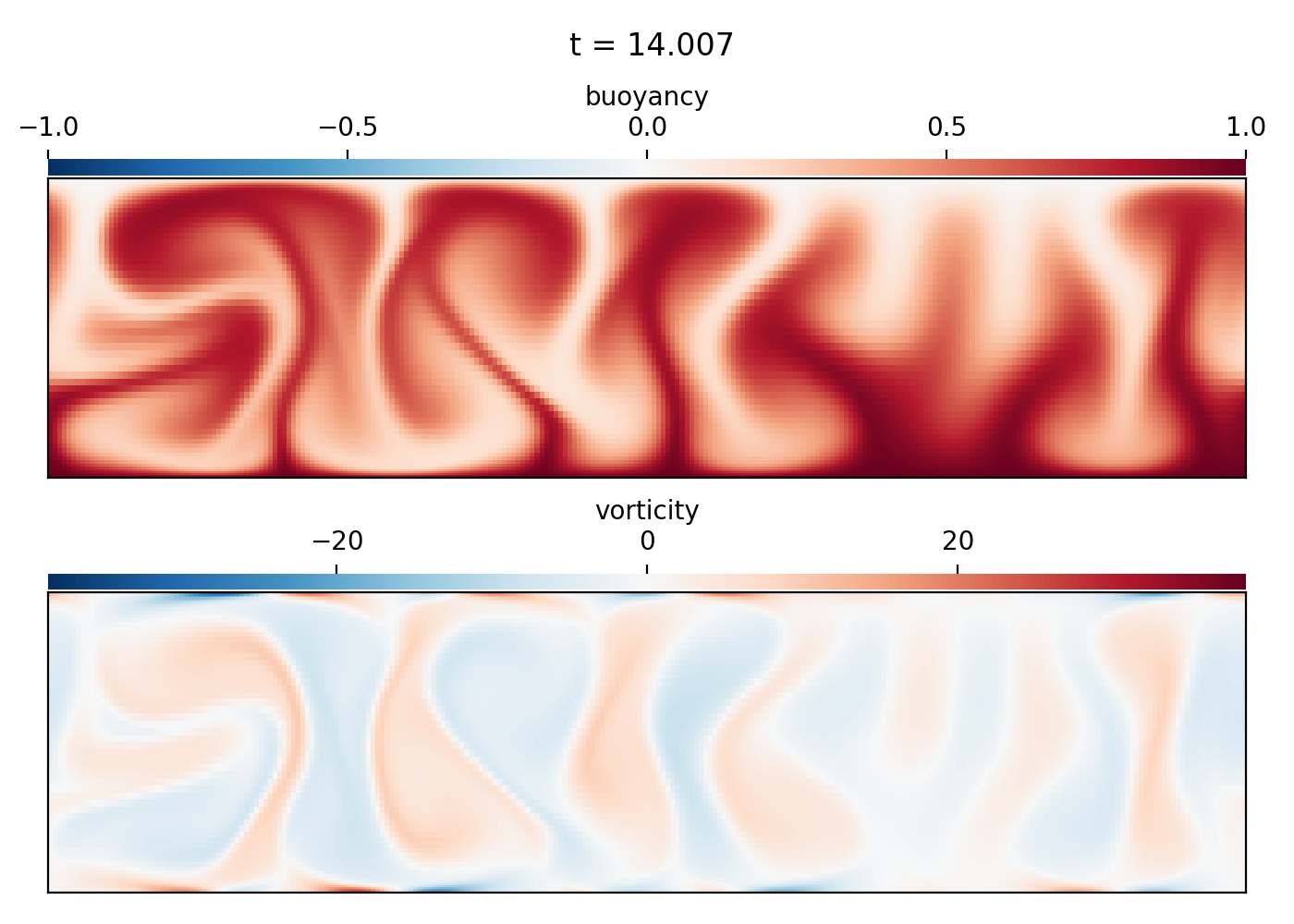# Rayleigh-Benard convection (2D IVP)

## Script

```"""
Dedalus script simulating 2D horizontally-periodic Rayleigh-Benard convection.
This script demonstrates solving a 2D Cartesian initial value problem. It can
be ran serially or in parallel, and uses the built-in analysis framework to save
data snapshots to HDF5 files. The `plot_snapshots.py` script can be used to
produce plots from the saved data. It should take about 5 cpu-minutes to run.

The problem is non-dimensionalized using the box height and freefall time, so
the resulting thermal diffusivity and viscosity are related to the Prandtl
and Rayleigh numbers as:

kappa = (Rayleigh * Prandtl)**(-1/2)
nu = (Rayleigh / Prandtl)**(-1/2)

For incompressible hydro with two boundaries, we need two tau terms for each the
velocity and buoyancy. Here we choose to use a first-order formulation, putting
one tau term each on auxiliary first-order gradient variables and the others in
the PDE, and lifting them all to the first derivative basis. This formulation puts
a tau term in the divergence constraint, as required for this geometry.

To run and plot using e.g. 4 processes:
\$ mpiexec -n 4 python3 rayleigh_benard.py
\$ mpiexec -n 4 python3 plot_snapshots.py snapshots/*.h5
"""

import numpy as np
import dedalus.public as d3
import logging
logger = logging.getLogger(__name__)

# Parameters
Lx, Lz = 4, 1
Nx, Nz = 256, 64
Rayleigh = 2e6
Prandtl = 1
dealias = 3/2
stop_sim_time = 50
timestepper = d3.RK222
max_timestep = 0.125
dtype = np.float64

# Bases
coords = d3.CartesianCoordinates('x', 'z')
dist = d3.Distributor(coords, dtype=dtype)
xbasis = d3.RealFourier(coords['x'], size=Nx, bounds=(0, Lx), dealias=dealias)
zbasis = d3.ChebyshevT(coords['z'], size=Nz, bounds=(0, Lz), dealias=dealias)

# Fields
p = dist.Field(name='p', bases=(xbasis,zbasis))
b = dist.Field(name='b', bases=(xbasis,zbasis))
u = dist.VectorField(coords, name='u', bases=(xbasis,zbasis))
tau_p = dist.Field(name='tau_p')
tau_b1 = dist.Field(name='tau_b1', bases=xbasis)
tau_b2 = dist.Field(name='tau_b2', bases=xbasis)
tau_u1 = dist.VectorField(coords, name='tau_u1', bases=xbasis)
tau_u2 = dist.VectorField(coords, name='tau_u2', bases=xbasis)

# Substitutions
kappa = (Rayleigh * Prandtl)**(-1/2)
nu = (Rayleigh / Prandtl)**(-1/2)
x, z = dist.local_grids(xbasis, zbasis)
ex, ez = coords.unit_vector_fields(dist)
lift_basis = zbasis.derivative_basis(1)
lift = lambda A: d3.Lift(A, lift_basis, -1)

# Problem
# First-order form: "div(f)" becomes "trace(grad_f)"
# First-order form: "lap(f)" becomes "div(grad_f)"
problem = d3.IVP([p, b, u, tau_p, tau_b1, tau_b2, tau_u1, tau_u2], namespace=locals())
problem.add_equation("integ(p) = 0") # Pressure gauge

# Solver
solver = problem.build_solver(timestepper)
solver.stop_sim_time = stop_sim_time

# Initial conditions
b.fill_random('g', seed=42, distribution='normal', scale=1e-3) # Random noise
b['g'] *= z * (Lz - z) # Damp noise at walls
b['g'] += Lz - z # Add linear background

# Analysis

# CFL
CFL = d3.CFL(solver, initial_dt=max_timestep, cadence=10, safety=0.5, threshold=0.05,
max_change=1.5, min_change=0.5, max_dt=max_timestep)

# Flow properties

# Main loop
startup_iter = 10
try:
logger.info('Starting main loop')
while solver.proceed:
timestep = CFL.compute_timestep()
solver.step(timestep)
if (solver.iteration-1) % 10 == 0:
max_Re = flow.max('Re')
logger.info('Iteration=%i, Time=%e, dt=%e, max(Re)=%f' %(solver.iteration, solver.sim_time, timestep, max_Re))
except:
logger.error('Exception raised, triggering end of main loop.')
raise
finally:
solver.log_stats()

```

## Output